Скачать презентацию Kick Off WB assignment Measurement and Dimensions

07fc671cd1ffe9a5ff941ac706c7dd1b.ppt

• Количество слайдов: 11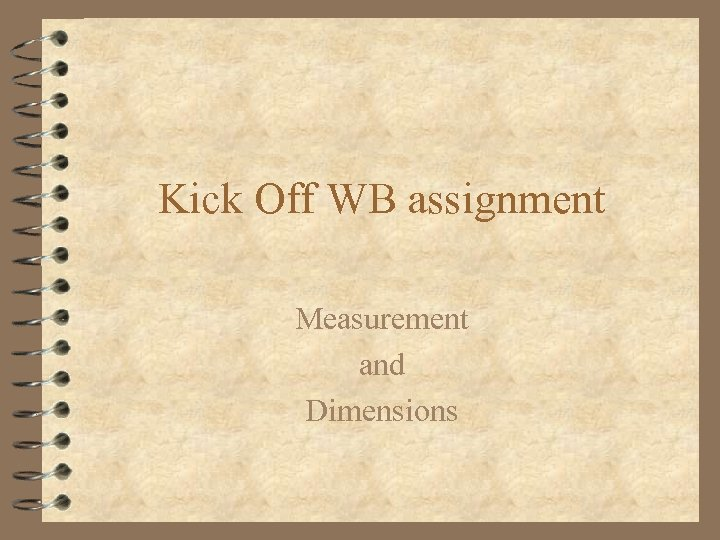Kick Off WB assignment Measurement and Dimensions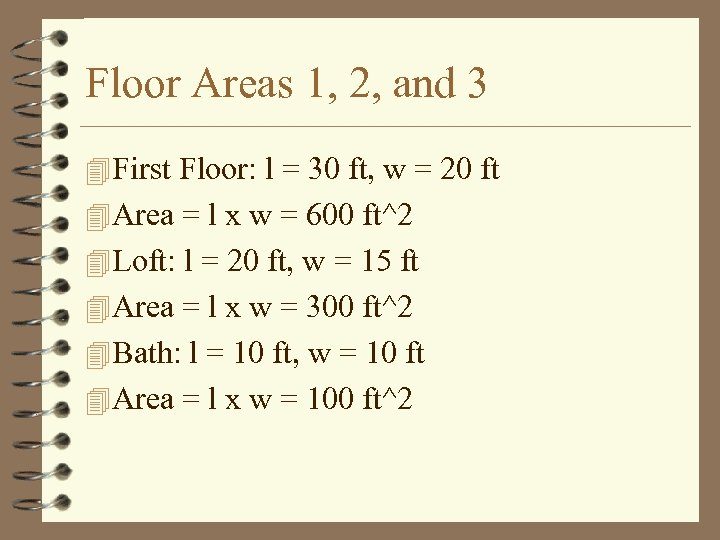Floor Areas 1, 2, and 3 4 First Floor: l = 30 ft, w = 20 ft 4 Area = l x w = 600 ft^2 4 Loft: l = 20 ft, w = 15 ft 4 Area = l x w = 300 ft^2 4 Bath: l = 10 ft, w = 10 ft 4 Area = l x w = 100 ft^2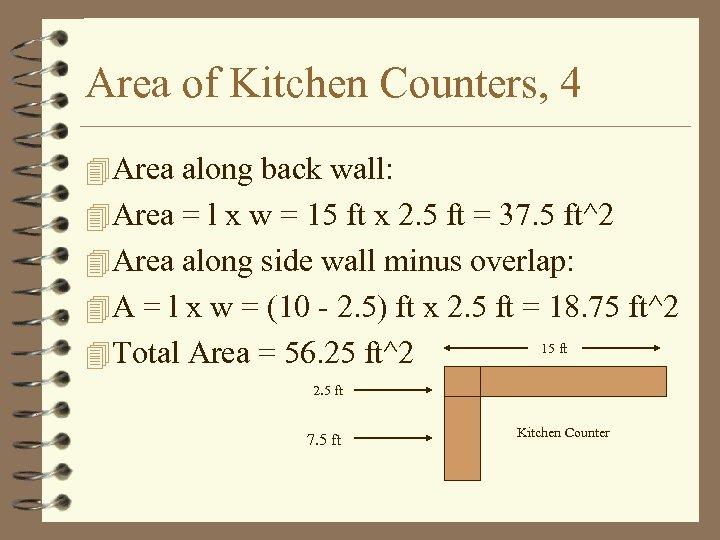Area of Kitchen Counters, 4 4 Area along back wall: 4 Area = l x w = 15 ft x 2. 5 ft = 37. 5 ft^2 4 Area along side wall minus overlap: 4 A = l x w = (10 - 2. 5) ft x 2. 5 ft = 18. 75 ft^2 4 Total Area = 56. 25 ft^2 15 ft 2. 5 ft 7. 5 ft Kitchen Counter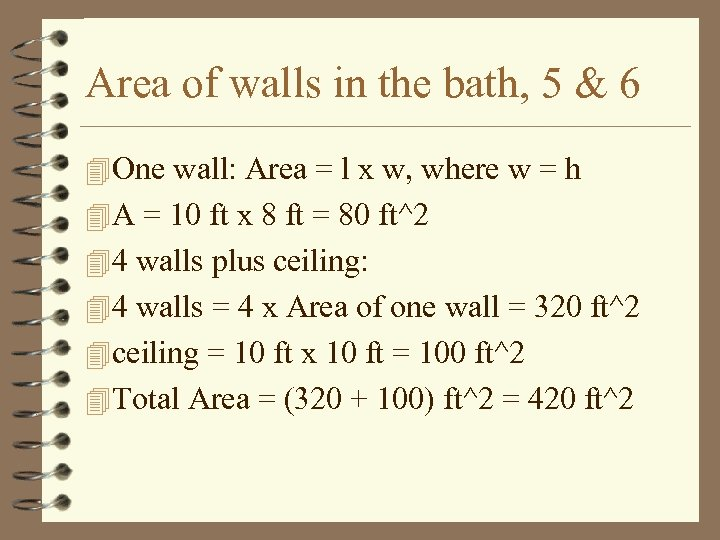Area of walls in the bath, 5 & 6 4 One wall: Area = l x w, where w = h 4 A = 10 ft x 8 ft = 80 ft^2 4 4 walls plus ceiling: 4 4 walls = 4 x Area of one wall = 320 ft^2 4 ceiling = 10 ft x 10 ft = 100 ft^2 4 Total Area = (320 + 100) ft^2 = 420 ft^2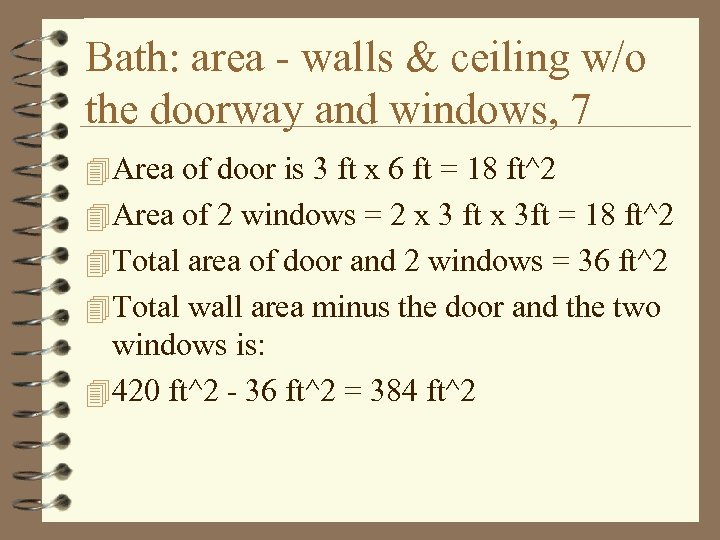Bath: area - walls & ceiling w/o the doorway and windows, 7 4 Area of door is 3 ft x 6 ft = 18 ft^2 4 Area of 2 windows = 2 x 3 ft x 3 ft = 18 ft^2 4 Total area of door and 2 windows = 36 ft^2 4 Total wall area minus the door and the two windows is: 4 420 ft^2 - 36 ft^2 = 384 ft^2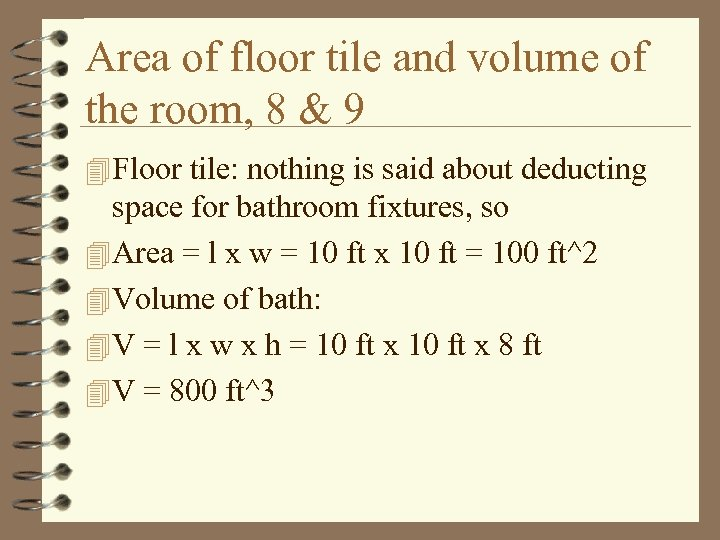Area of floor tile and volume of the room, 8 & 9 4 Floor tile: nothing is said about deducting space for bathroom fixtures, so 4 Area = l x w = 10 ft x 10 ft = 100 ft^2 4 Volume of bath: 4 V = l x w x h = 10 ft x 8 ft 4 V = 800 ft^3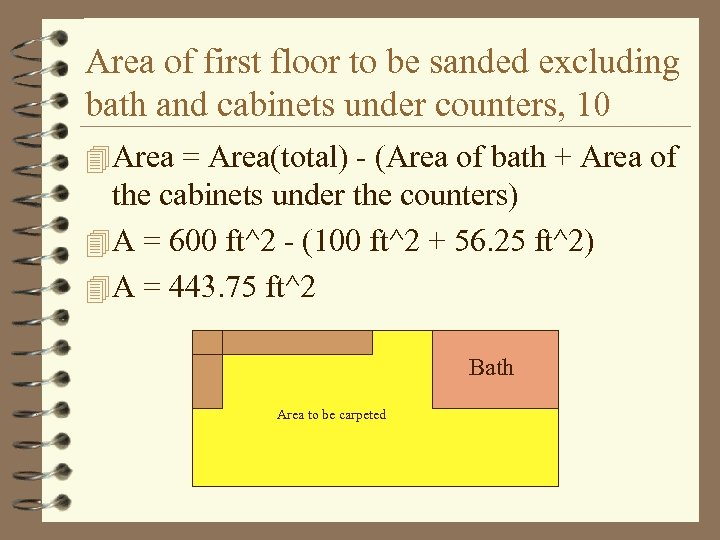Area of first floor to be sanded excluding bath and cabinets under counters, 10 4 Area = Area(total) - (Area of bath + Area of the cabinets under the counters) 4 A = 600 ft^2 - (100 ft^2 + 56. 25 ft^2) 4 A = 443. 75 ft^2 Bath Area to be carpeted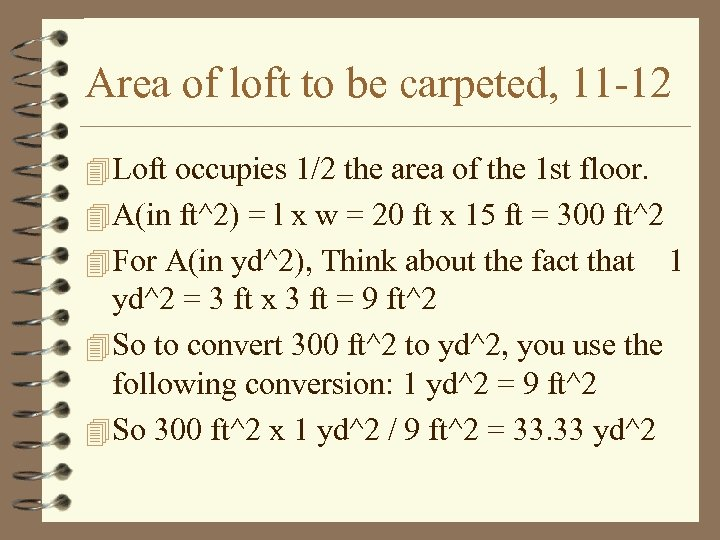Area of loft to be carpeted, 11 -12 4 Loft occupies 1/2 the area of the 1 st floor. 4 A(in ft^2) = l x w = 20 ft x 15 ft = 300 ft^2 4 For A(in yd^2), Think about the fact that yd^2 = 3 ft x 3 ft = 9 ft^2 4 So to convert 300 ft^2 to yd^2, you use the following conversion: 1 yd^2 = 9 ft^2 4 So 300 ft^2 x 1 yd^2 / 9 ft^2 = 33. 33 yd^2 1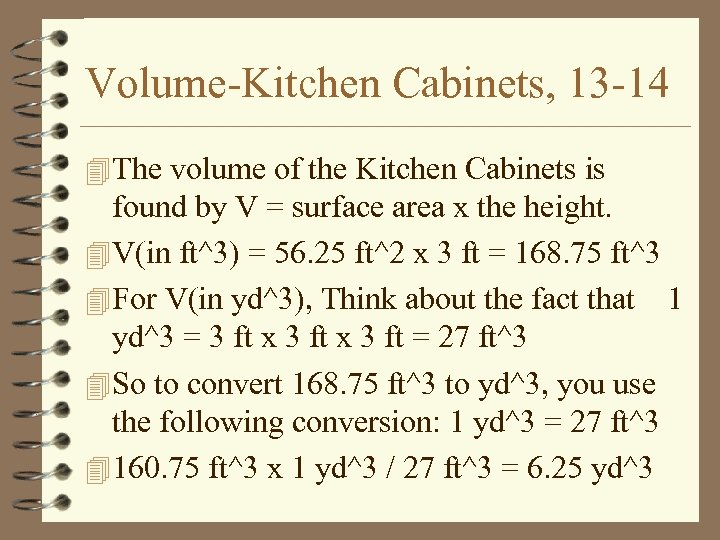Volume-Kitchen Cabinets, 13 -14 4 The volume of the Kitchen Cabinets is found by V = surface area x the height. 4 V(in ft^3) = 56. 25 ft^2 x 3 ft = 168. 75 ft^3 4 For V(in yd^3), Think about the fact that 1 yd^3 = 3 ft x 3 ft = 27 ft^3 4 So to convert 168. 75 ft^3 to yd^3, you use the following conversion: 1 yd^3 = 27 ft^3 4 160. 75 ft^3 x 1 yd^3 / 27 ft^3 = 6. 25 yd^3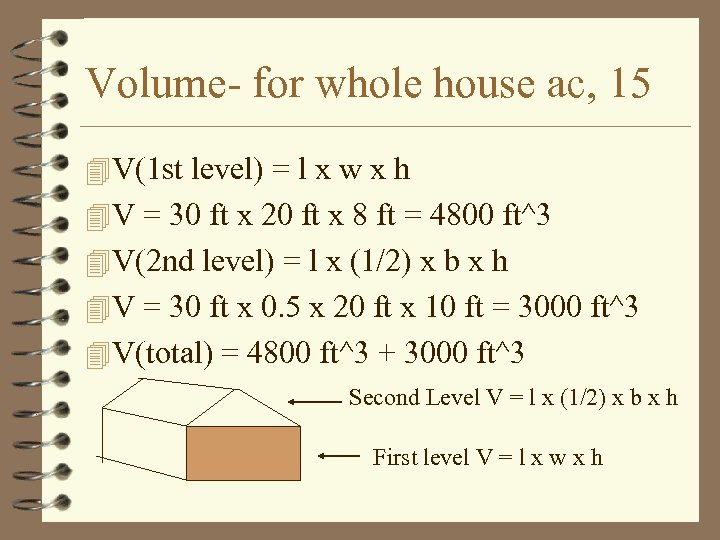Volume- for whole house ac, 15 4 V(1 st level) = l x w x h 4 V = 30 ft x 20 ft x 8 ft = 4800 ft^3 4 V(2 nd level) = l x (1/2) x b x h 4 V = 30 ft x 0. 5 x 20 ft x 10 ft = 3000 ft^3 4 V(total) = 4800 ft^3 + 3000 ft^3 Second Level V = l x (1/2) x b x h First level V = l x w x h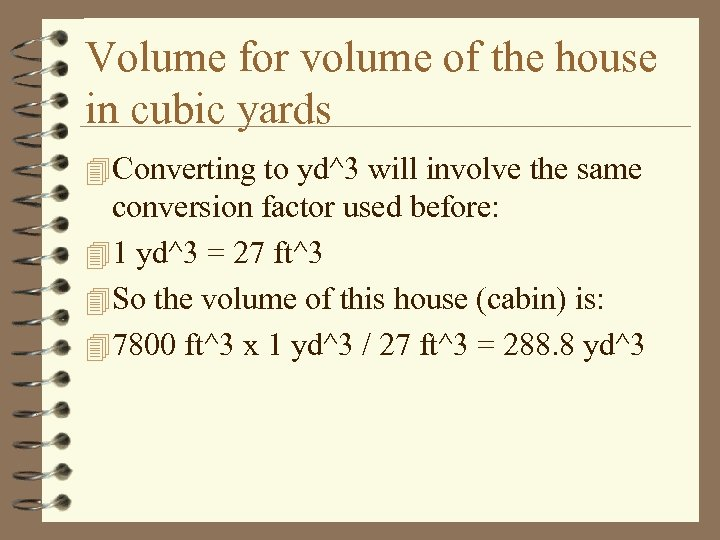Volume for volume of the house in cubic yards 4 Converting to yd^3 will involve the same conversion factor used before: 4 1 yd^3 = 27 ft^3 4 So the volume of this house (cabin) is: 4 7800 ft^3 x 1 yd^3 / 27 ft^3 = 288. 8 yd^3